## Tuesday, October 19, 2010

### Piecewise-linear Functions in Math Programs

How to handle a piecewise-linear function of one variable in an optimization model depends to a large extent on whether it represents an economy of scale, a diseconomy of scale, or neither. The concepts apply generally, but in this post I'll stick to cost functions (where smaller is better, unless you are the federal government) for examples; application to things like productivity measures (where more is better) requires some obvious and straightforward adjustments. Also, I'll stick to continuous functions today.

Let's start with a diseconomy of scale. On the cost side, this is something that grows progressively more expensive at the margin as the argument increases. Wage-based labor is a good example (where, say, time-and-a-half overtime kicks in beyond some point, and double-time beyond some other point). A general representation for such a function is $f(x)=a_{i}+b_{i}x\mbox{ for }x\in[d_{i-1},d_{i}],\: i\in\{1,\ldots,N\}$ where $d_{0}=-\infty$ and $d_{N}=+\infty$ are allowed and where the following conditions must be met: \begin{eqnarray*}d_{i-1} & < & d_{i}\:\forall i\in\{1,\ldots,N\}\\b_{i-1} & < & b_{i}\:\forall i\in\{2,\ldots,N\}\\a_{i-1}+b_{i-1}d_{i-1} & = & a_{i}+b_{i}d_{i-1}\:\forall i\in\{2,\ldots,N\}.\end{eqnarray*}The first condition just enforces consistent indexing of the intervals, the second condition enforces the diseconomy of scale, and the last condition enforces continuity. The diseconomy of scale ensures that $f$ is a convex function, as illustrated in the following graph.
Letting $f_{i}(x)=a_{i}+b_{i}x$, we can see from the graph that $f$ is just the upper envelope of the set of functions $\{f_{1},\ldots,f_{N}\}$ (easily verified algebraically). To incorporate $f(x)$ in a mathematical program, we introduce a new variable $z$ to act as a surrogate for $f(x)$, and add the constraints$z\ge a_{i}+b_{i}x\;\forall i\in\{1,\ldots,N\}$ to the model. Anywhere we would use $f(x)$, we use $z$ instead. Typically $f(x)$ is either directly minimized in the objective or indirectly minimized (due to its influence on other objective terms),
and so the optimal solution will contain the minimum possible value of $z$ consistent with the added constraints. In other words, one of the constraints defining $z$ will be binding. On rare occasions this will not hold (for instance, cost is relevant only in satisfying a budget, and the budget limit is quite liberal). In such circumstances, the optimal solution may have $z>f(x)$, but that will not cause an incorrect choice of $x$, and the value of $z$ can be manually adjusted to $f(x)$ after the fact.

Now let's consider an economy of scale, such as might occur when $x$ is a quantity of materials purchases, $f(x)$ is the total purchase cost, and the vendor offers discounts for larger purchases. The marginal rate of change of $f$ is now nonincreasing as a function of $x$, and as a result $f$ is concave, as in the next graph.
Now $f$ is the lower envelope of $\{f_{1},\ldots,f_{N}\}$. We define the segments as before, save with the middle condition reversed to $b_{i-1}>b_{i}\:\forall i\in\{2,\dots,N\}$. We cannot simply add constraints of the form $z\le a_{i}+b_{i}x$ if we are (directly or indirectly) minimizing $z$, since $z=-\infty$ would be optimal (or, if we asserted a finite lower bound for $z$, the lower bound would automatically be optimal). In the presence of an economy of scale, we are forced to add binary variables to the formulation, either directly or indirectly. The easiest way to do this is to use a type 2 specially ordered set (SOS2) constraint, provided that the solver (and any modeling language/system) understand SOS2, and provided that $d_{0}$ and $d_{N}$ are finite. Introduce new (continuous) variables $\lambda_{0},\ldots,\lambda_{N}\ge0$ along with $z$, and introduce the following  constraints:\begin{eqnarray*}\lambda_{0}+\cdots+\lambda_{N} & = & 1 \\ \lambda_{0}d_{0}+\cdots+\lambda_{N}d_{N} & = & x\\ \lambda_{0}f(d_{0})+\cdots+\lambda_{N}f(d_{N}) & = & z.\end{eqnarray*} Also communicate to the solver that $\{\lambda_{0},\ldots,\lambda_{N}\}$ is an SOS2 set. The SOS2 constraint says that at most two of the $\lambda$ variables can be nonzero, and that if two are nonzero they must be consecutive. Suppose that $\lambda_{i}$ and $\lambda_{i+1}$ are positive and all others are zero. Then the new constraints express $x$ as a convex combination of $d_{i}$ and $d_{i+1}$ (with weights $\lambda_{i}$ and $\lambda_{i+1}=1-\lambda_{i}$), and express $z=f(x)$ as a convex combination of $f(d_{i})$ and $f(d_{i+1}$) using the same weights.

If SOS2 constraints are not an option, then binary variables have to be added explicitly. Let $\delta_{i}\in\{0,1\}$ designate whether $x$ belongs to the interval $[d_{i-1},d_{i}]$ ($\delta_{i}=1$) or not ($\delta_{i}=0$). Add the following constraints:\begin{eqnarray*}\delta_{1}+\cdots+\delta_{N} & = & 1\\ x & \le & d_{i}+M(1-\delta_{i})\:\forall i\in\{1,\ldots,N\}\\ x & \ge & d_{i-1}-M(1-\delta_{i})\:\forall i\in\{1,\ldots,N\}\\ z & \ge & a_{i}+b_{i}x-M(1-\delta_{i})\:\forall i\in\{1,\ldots,N\}\end{eqnarray*} where $M$ is a suitably large constant. (This formulation will again require that $d_{0}$ and $d_{N}$ be finite.) The first constraint says that $x$ must belong to exactly one interval. If $\delta_{j}=1$, the second and third constraints force $d_{j-1}\le x\le d_{j}$ and are vacuous for all $i\neq j$ (assuming $M$ is correctly chosen). The final constraint forces $z\ge f_{j}(x)$ and is again vacuous for all $i\neq j$. Since $z$ represents something to be minimized, as before we expect $z=f_{j}(x)$ in almost all cases, and can manually adjust it in the few instances were the optimal solution contains padding.

1.This comment has been removed by the author.

2.Thank you for all these details. Do you recommend any free software to handle SOS2?. Regards

3.I believe that CBC (which you'll find at http://www.coin-or.org/projects/) has SOS2 support. I'm not sure about the other COIN MIP solvers listed there; some of them may as well. If you are a college/university professor, you can get free licenses to CPLEX and Gurobi. CPLEX supports SOS2, and I'm pretty sure Gurobi does as well.

4.Dear Paul,

It's a excellent post. I have academic version of cplex. As well as I have Ampl. I am requesting you to give me the foloowing information. How can I declare a SOS2 type variable using AMPL? I am new in this area.

Regards,

Dewan

5.If the reason to use SOS2 constraints is to express piecewise-linear constraints, AMPL has its own notation for such constraints (involving lists of breakpoints and slopes), and my understanding is that SOS2-savvy solvers such as CPLEX will automatically use SOS2 to implement those constraints. The notation is covered in the AMPL book (chapter 17 maybe -- I don't have a current edition).

If for some reason you want or need to express SOS2 constraints directly, there's an example late in this thread: https://groups.google.com/forum/?fromgroups#!topic/ampl/Mo8F0dvHWms. You have to define two suffixes, sosno and ref, and apply them to the variables in the special ordered sets. The sosno suffix identifies the SOS to which the variable belongs, and if I recall correctly (a big "if" in this case) the sign of the sosno determines the type of SOS (positive -> SOS1, negative -> SOS2). For SOS2, the ref suffix indicates the sequencing of the variables. For SOS1, I believe the ref suffix assigns weights (but, again, my memory is a bit shaky).

6.Dear Paul,

Thank you very much for your quick reply. The info you described above is surely helpful for me to work with SOS2 constraints.

Regards,

Dewan

7.Dear Paul,

Good afternoon.

I have used the concept of "economy of scale" in my project. And its giving optimal results for my project. Before going to defend my project, I am searching a paper or document to proof the optimality of "economy of scale" concept that you discussed above. I will be very much grateful to you, if you provide me the link some papers.

Best regards,

Dewan

8.@Dewan,

I'm not sure exactly what you want, but I think you might find it in the book "Model Building in Mathematically Programming" by H. Paul Williams. I don't know what the current edition is, but in the fourth edition you might want to look in chapter 7. (The index helpfully includes an entry for "economies of scale".)

9.Good morning Paul,

Thank you very much for the information.

I requested you about "proof of global optimality and convergence" of a concave objective function like "economy of scale" function. Currently I am working to piece-wise linear approximate a concave objective function of one variable that contains an abs(sin(X)), i.e., a quadratic concave cost function of one variable along with absolute valve of SIN(X). The "economy of scale" concept that you described above is working fine for my piece-wise linear approximating of a concave objective function with ABS(SIN(X)) after applying the concept of "economy of scale". And I am getting global optimal solution but I don't how I can proof that it gives a global optimal solution. If I defend my model without knowing that reviewers can ask me what the proof of global optimality and convergence of the concave cost function.

I found "proof of global optimality and convergence" of non-convex cost function but I could not find any proof for concave cost function.

I will read the chapter 7 of the book. Thanks for the info.

Best regards,

Dewan

10.@Dewan: I think there are two issues here: whether the solution to the MILP model is actually a global optimum of the pw-linear approximation; and whether the global optimum of the pw-linear approximation is adequately good as an approximation to the optimum of the original problem. I don't know off-hand a published proof of the first, but that's because it is a rather trivial proof. Suppose that the MILP optimum is not optimal for the pw-linear approximation. Then there exists a solution feasible x* in the pw-linear problem with a strictly superior objective value. Simply select the correct values for the binary variables (i.e., the ones that select the segment containing x*), demonstrate that with those values for the binaries and x = x* you have a feasible solution to the MILP, and assert a contradiction to the optimality of the MILP solution.

As far as the second point goes, if the issue is how close the MILP objective is to optimal, you just need to compute the worst difference between the MILP objective and your original objective. If the issue is how close the MILP solution x* is to the true global solution (say, in Euclidean norm), that's a bit trickier. You could conceivably be on the opposite side of the feasible region from the true optimal x and be quite close in objective value.

11.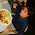Is there any way to make the following cost function into LMP using AMPL /OPL studio etc.

var distance_travelled[route];

Objective is
minimize totalcost: sum{r in routes} cost[r];

where cost[r] is :-

1000 unit if distance_travelled[r] is 0 to 25km
2000 unit if distance_travelled[r] is 25 to 50km
3500 unit if distance_travelled[r] is 50 to 100km
and 40unit/KM if distance_travelled[r] is more than 100 Km

Thanks
Arun Lila

12.AMPL supports SOS2 declarations, so you can use an approach I just posted elsewhere (http://orinanobworld.blogspot.com/2012/08/step-functions-and-mixed-functions.html). You might also look at the related posts at the bottom of that one, particularly the last one. AMPL also has its own syntax for piecewise-linear objects, which is the equivalent of what I mention in the bottom link.

13.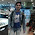Dear Mr Paul,
Would you please suggest me some software or package like AMPl which will allow me to convert a non linear function into a piece wise linearly approximated function using SOS2. I have already installed GUROBI to solve some simple non linear functions using SOS2, but gurobi is a solver while i need some modelling language which can allow me to convert my nonlinear function into piecewise linear function. Also would you please tell me that how to convert cos(tan inverse of w) into piecewise function using SOS2, your help will be much appreciated .

1.Just to be clear, you cannot convert an arbitrary nonlinear function into a piecewise-linear function; you can only approximate it with a piecewise-linear function.

AMPL supports piecewise-linear functions, and I believe other modeling languages do as well. I don't know of any modeling language that will compute the parameters (breakpoints, slopes) of a piecewise-linear approximation to a nonlinear function for you. You will have to do that yourself. AMPL will handle the conversion of a piecewise-linear function to a representation suitable for an integer linear program if necessary. (I don't know if it uses an SOS2 representation or some other approach.)

That said, you might be better off using a nonlinear program solver, such as KNITRO (which is supported by a number of modeling languages, including AMPL).

To approximate your specific function, pick values for w, compute the function at those points, and do a linear interpolation between the points. Once you have an idea of which range is likely to contain the optimal w, refine the approximation by adding more interpolation points near that w value, solve again, and iterate until you are happy with the answer.

14.Thank you very much Sir...i will try your suggestions

15.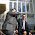Thanks so much for the material, I have a function that is f(y)=a*y^b, where a is a positive number and b<1 (a concave function) to represent the economies of scale. I would like to approximate the function by a set of piecewise linear functions who describe the function using the lower envelope of tangent lines. What is the linearization of f(y).

1.In order to stick to my notation in the body of the post, let me change yours a bit: we'll call your variable x rather than y, and your concave function g(x) = h*x^p with h > 0 and 0 < p < 1. Your first step is to discretize the domain of x by choosing break points x_1 < x_2 < ... < x_{N+1}. The number and placement of the break points are up to you. You then need to calculate linear functions f_n(x) = a_n + b_n*x that are tangent to g at x = x_n, which is straightforward: b_n = g'(x_n), and a_n = g(x_n) - b_n*x_n. Next, find the intersection points d_1 < ... < d_N such that f_n(d_n) = f_{n+1}(d_n). You'll need to add endpoints d_0 and d_{N+1} to capture the full domain of x. Now you just follow the economy of scale approach I used above, with f() the lower envelope of the f_n().

Once you've solved the model and gotten x = x*, you might want to reduce the domain of x to a tighter interval around x* and repeat the process (discretizing the new domain, computing new tangents, ...) to refine the solution a bit. Basically, the question comes down to whether the difference between the envelope value f(x*) and the true value g(x*) is worth getting excited about.

2.Thanks so much Prof. Paul,

16.Hello,
Thanks for your post. I have two questions, I appreciate if you can give me some hints.
1- I need to write the steps in discontinuous piecewise linear functions (stepwise) in a decreasing order. For example the first step is 1 but the second should decrease to 0.8. Can I define negative steps like this:

piecewise{ 0->7904; -0.1->7904; 0->11856; -0.1->11856; 0}(7903,1) X * y

2- in the sample function above, lets say X is a decision variable and y is a parameter. I dont want the value of piecewise function to be selected based on X * y but only X. does using a parenthesis make this distinction? or the function below is equal to the one above?

(piecewise{ 0->7904; -0.1->7904; 0->11856; -0.1->11856; 0}(7903,1) X) * y

Thank you so much in advance
Helia

1.This seems to be a syntax question, but you did not indicate what language you are using. It looks close to OPL, but I'm not sure it is valid OPL syntax. In any case, I don't know much about OPL, so if that is what you are using, you might want to try the discussion forum for it: https://www.ibm.com/developerworks/community/forums/html/forum?id=11111111-0000-0000-0000-000000002053.

2.Dear Mr Rubin,

Yes, I write OPL Language. I was just going to write a reply, because I have found an answer to one question. I should use stepwisefunction; then I dont need to worry for increasing or decreasing steps. The following link is a very helpful document (for those who might be interested) particularly page 71:

http://www.ensta-paristech.fr/~diam/ro/online/ilog/cplex124_pdfs/opl_langref.pdf

For my second question though I have not yet figured out if the parenthesis helps or not. I will check the link you have provided.

Many thanks,
Helia

17.Hello Paul,
I'm doing some modeling using Gurobi, and I encounter a problem (Q is not psd)when I tried to set a quadratic constraint like this : cx - s*x = b, I wonder if I can use SOS 2 to solve my problem by transforming cx-s*x = b to s(x) = b/(c-x) , then use sos 2 to linearize it ? Can I approximate the function by a set of piecewise linear function? Then what is the linearization of s(x).? Or is there any other way to solve it ?
Thank you!

Thank you !

Thank yo

1.I answered this on the other post where you asked it. Meanwhile, you might find this informative: http://www.urbandictionary.com/define.php?term=cross-posting.

2.18.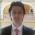Dear Dr. Rubin,

Thank you so much for the post. How can I cite this work in my study?

1.You're welcome. The content is "general knowledge", nothing original, so you do not need to cite it for purposes of acknowledging authorship. If you want to cite it to demonstrate that someone other than you actually said it, the citation style will depend on the publication (journal?) to which you are submitting. The Wikipedia lists a variety of ways to cite Wikipedia pages (https://en.wikipedia.org/wiki/Wikipedia:Citing_Wikipedia); you should be able to adapt one to this blog.

19.Hi,
Is there any possible link or tutorial that highlights how to use SOS2 with CBC or CyLP?

1.I don't use either CBC or CyLP, so I'm afraid I cannot point you directly to a tutorial or example code. There is a mailing list for CBC, though, which you can access from a link in the bottom of their project page (https://projects.coin-or.org/Cbc). The archives don't have a search feature, but Google can help you out. For instance, you could try the search query "sos2 site:https://list.coin-or.org/pipermail/cbc/".

2.Just found out that there is no mailing list for CyLP. The issue tracker on the project's GitHub page serves that role.

20.Thank you so much for the prompt replies, could you recommend any python module (that provides an interface to a solver) which could allow handling of SOS2 ?

1.Sorry, no; I don't work with Python.

21.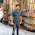Dear Sir
I have function
if c A(c) = 1;elseif (c1 A(c) = 1- eq;elseif(c>c2) =>A(c) = 0;
what would be my breakpoints if i want to solve this by piece wise linear?

1.Sorry, your condition does not make any sense. Might it be missing a few symbols? If you know LaTeX, you can write LaTeX math notation bracketed by dollar signs. When you post the comment, you won't see the math output, but after refreshing the page you should.

22.Very useful post. I used it to guide the construction of a income tax calculation and it works great. I then tried to use it for calculation of capital gains taxes but this does not work. Basically I created the same piecewise linear tax function and then tried ctg >= f(ti+cg) - f(ti) where cgt is the capital gains tax, f() is the piecewise linear function, ti is taxable income and cg is capital gains. What happens is that f(ti) rises to match f(ti+cg) so cgt becomes zero. I don't know how to correct this or how to rewrite the capital gains tax function to get around this. Any ideas?
Thanks much,

1.I'm not sure I'm following this, but my first guess would be that you might be minimizing ctg rather than minimizing total tax. If I were filling out a tax return and focused only on minimizing capital gains tax, I would declare all my income as "ordinary". That would give me not capital gains tax (but would have me paying more tax overall, since I just passed up the lower CG rate in favor of the higher ordinary rate).

23.Sorry for not being more clear. The object function I want is to maximize spendable dollars. Call this 'spendable.' Then I have another constraint that takes income sources with their various type of taxes and other expenses to define s. The model is to create a plan for using retirement funds optimally. So as it maximizes after tax spendable money the constraint defining s includes these income(s) minus their taxes. So, yes, the lower the tax the higher the spendable money.

maximize spendable
s.t.
401k Withdrawal + Investment Account Withdrawal - Income tax - CapGainsTax <= spendable
Income tax >= 7 linear functions for the income tax brackets x=taxable income
temp1 >= 3 linear functions for the capital gains brackets x=taxable income + capital gains
temp2 >= 3 linear functions for the capital gains brackets x=taxable income
CapGainsTax >= temp1 - temp2

I hope that is more clear.
Thanks,

1.I assume that first constraint was supposed to be == or >= rather than <=; otherwise the model is unbounded. Assuming that, spendable increases as CG tax decreases, and your model lets you have zero CG tax by setting temp2 equal to temp1. I suspect you need to tie temp2 to income tax in a way that discourages padding temp2, but that is an accounting question and I am (blissfully!) ignorant of accounting.

24.Thanks Paul.

When I include temp2 (capital gains tax associated with taxable income) in the objective function the cgt constraint works as I want but it has the, for me, unintended side effect of causing the model to minimize income tax which is slightly different from maximizing spendable. The minimization gives unwanted transfers of money from 401k to investment account. All that does lower the taxes and increase the object function while slightly lowering spendable.

I think I will try to convert the capital gains function from 2d to 3d and having piecewise linear plains to represent the function. Have you ever seen this done? know of any examples?

Thanks Much,

1.I've seen pw-linear functions of two arguments, but not in a while, and I can't point to any examples.

25.OK, again thanks much. Very helpful and I learned something new :-)

26.Hello Professor Rubin,
I am studying on probabilistic chance constraints. My objective function is in the shape you mentioned here,

https://www.quora.com/How-can-I-solve-a-constrained-optimization-problem-where-the-objective-function-is-sum-of-a-linear-expression-and-an-exponential-of-linear-expression

I understood additional constraint but I could not understand how I will modify the objective function ? How can I solve this problem ,with which solver ?

Any help is appreciated

Thank you so much

1.You would maximize $b'x + \sum_{i=0}^N e^{W_i} y_i$, which is linear in $x$ and $y$. Any integer linear programming solver that recognizes SOS2 constraints could be used.

Due to intermittent spamming, comments are being moderated. If this is your first time commenting on the blog, please read the Ground Rules for Comments. In particular, if you want to ask an operations research-related question not relevant to this post, consider asking it on Operations Research Stack Exchange.